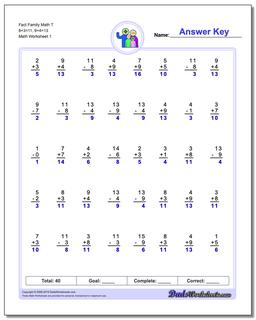One Dad. Four daughters. 9,251 worksheets... and counting!Fact Family Math T 8+3=11, 9+4=13

PropertyValue
DescriptionFact Family Math T 8+3=11, 9+4=13: These 40 problem addition and subtraction fact family worksheets are perfect for either quick practice or a one-minute timed test. Be sure to check out the stand-alone addition worksheets and subtraction worksheets for help on specific operations.
Resource TypeWorksheet